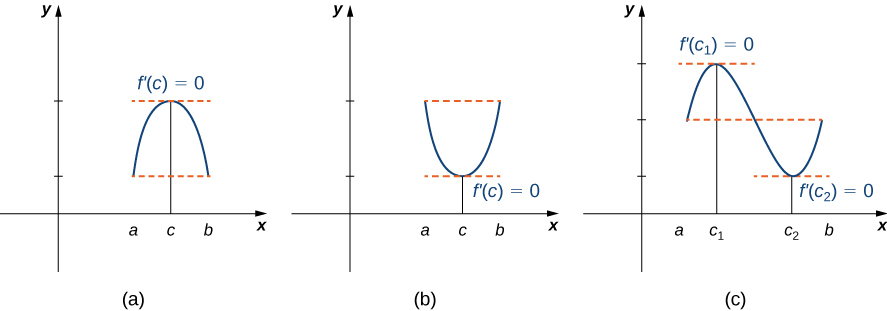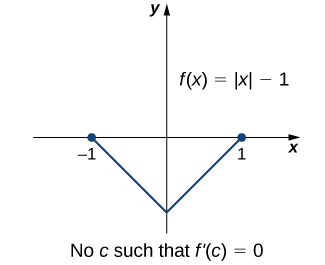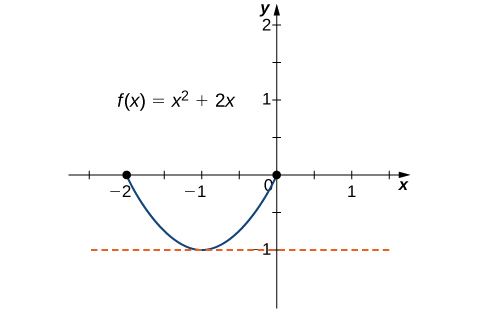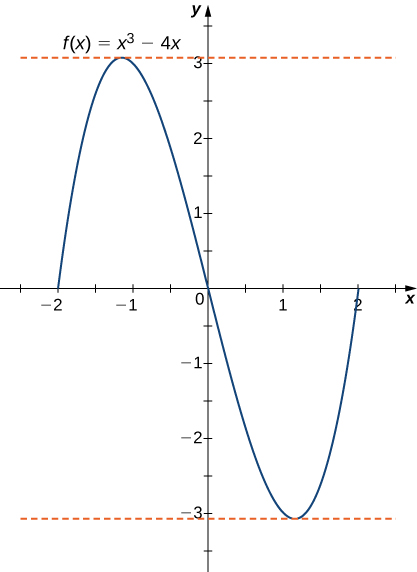# 4.4 The mean value theorem

 Page 1 / 7
• Explain the meaning of Rolle’s theorem.
• Describe the significance of the Mean Value Theorem.
• State three important consequences of the Mean Value Theorem.

The Mean Value Theorem is one of the most important theorems in calculus. We look at some of its implications at the end of this section. First, let’s start with a special case of the Mean Value Theorem, called Rolle’s theorem.

## Rolle’s theorem

Informally, Rolle’s theorem states that if the outputs of a differentiable function $f$ are equal at the endpoints of an interval, then there must be an interior point $c$ where $f\prime \left(c\right)=0.$ [link] illustrates this theorem.If a differentiable function f satisfies f ( a ) = f ( b ) , then its derivative must be zero at some point(s) between a and b .

## Rolle’s theorem

Let $f$ be a continuous function over the closed interval $\left[a,b\right]$ and differentiable over the open interval $\left(a,b\right)$ such that $f\left(a\right)=f\left(b\right).$ There then exists at least one $c\in \left(a,b\right)$ such that $f\prime \left(c\right)=0.$

## Proof

Let $k=f\left(a\right)=f\left(b\right).$ We consider three cases:

1. $f\left(x\right)=k$ for all $x\in \left(a,b\right).$
2. There exists $x\in \left(a,b\right)$ such that $f\left(x\right)>k.$
3. There exists $x\in \left(a,b\right)$ such that $f\left(x\right)

Case 1: If $f\left(x\right)=0$ for all $x\in \left(a,b\right),$ then $f\prime \left(x\right)=0$ for all $x\in \left(a,b\right).$

Case 2: Since $f$ is a continuous function over the closed, bounded interval $\left[a,b\right],$ by the extreme value theorem, it has an absolute maximum. Also, since there is a point $x\in \left(a,b\right)$ such that $f\left(x\right)>k,$ the absolute maximum is greater than $k.$ Therefore, the absolute maximum does not occur at either endpoint. As a result, the absolute maximum must occur at an interior point $c\in \left(a,b\right).$ Because $f$ has a maximum at an interior point $c,$ and $f$ is differentiable at $c,$ by Fermat’s theorem, $f\prime \left(c\right)=0.$

Case 3: The case when there exists a point $x\in \left(a,b\right)$ such that $f\left(x\right) is analogous to case 2, with maximum replaced by minimum.

An important point about Rolle’s theorem is that the differentiability of the function $f$ is critical. If $f$ is not differentiable, even at a single point, the result may not hold. For example, the function $f\left(x\right)=|x|-1$ is continuous over $\left[-1,1\right]$ and $f\left(-1\right)=0=f\left(1\right),$ but $f\prime \left(c\right)\ne 0$ for any $c\in \left(-1,1\right)$ as shown in the following figure.Since f ( x ) = | x | − 1 is not differentiable at x = 0 , the conditions of Rolle’s theorem are not satisfied. In fact, the conclusion does not hold here; there is no c ∈ ( −1 , 1 ) such that f ′ ( c ) = 0 .

Let’s now consider functions that satisfy the conditions of Rolle’s theorem and calculate explicitly the points $c$ where $f\prime \left(c\right)=0.$

## Using rolle’s theorem

For each of the following functions, verify that the function satisfies the criteria stated in Rolle’s theorem and find all values $c$ in the given interval where $f\prime \left(c\right)=0.$

1. $f\left(x\right)={x}^{2}+2x$ over $\left[-2,0\right]$
2. $f\left(x\right)={x}^{3}-4x$ over $\left[-2,2\right]$
1. Since $f$ is a polynomial, it is continuous and differentiable everywhere. In addition, $f\left(-2\right)=0=f\left(0\right).$ Therefore, $f$ satisfies the criteria of Rolle’s theorem. We conclude that there exists at least one value $c\in \left(-2,0\right)$ such that $f\prime \left(c\right)=0.$ Since $f\prime \left(x\right)=2x+2=2\left(x+1\right),$ we see that $f\prime \left(c\right)=2\left(c+1\right)=0$ implies $c=-1$ as shown in the following graph.This function is continuous and differentiable over [ −2 , 0 ] , f ′ ( c ) = 0 when c = −1 .
2. As in part a. $f$ is a polynomial and therefore is continuous and differentiable everywhere. Also, $f\left(-2\right)=0=f\left(2\right).$ That said, $f$ satisfies the criteria of Rolle’s theorem. Differentiating, we find that $f\prime \left(x\right)=3{x}^{2}-4.$ Therefore, $f\prime \left(c\right)=0$ when $x=\text{±}\frac{2}{\sqrt{3}}.$ Both points are in the interval $\left[-2,2\right],$ and, therefore, both points satisfy the conclusion of Rolle’s theorem as shown in the following graph.For this polynomial over [ −2 , 2 ] , f ′ ( c ) = 0 at x = ± 2 / 3 .

any genius online ? I need help!!
Pina
need to learn polynomial
Zakariya
i will teach...
nandu
I'm waiting
Zakariya
evaluate the following computation (x³-8/x-2)
teach me how to solve the first law of calculus.
what is differentiation
f(x) = x-2 g(x) = 3x + 5 fog(x)? f(x)/g(x)
fog(x)= f(g(x)) = x-2 = 3x+5-2 = 3x+3 f(x)/g(x)= x-2/3x+5
diron
pweding paturo nsa calculus?
jimmy
how to use fundamental theorem to solve exponential
find the bounded area of the parabola y^2=4x and y=16x
what is absolute value means?
Chicken nuggets
Hugh
🐔
MM
🐔🦃 nuggets
MM
(mathematics) For a complex number a+bi, the principal square root of the sum of the squares of its real and imaginary parts, √a2+b2 . Denoted by | |. The absolute value |x| of a real number x is √x2 , which is equal to x if x is non-negative, and −x if x is negative.
Ismael
find integration of loge x
find the volume of a solid about the y-axis, x=0, x=1, y=0, y=7+x^3
how does this work
Can calculus give the answers as same as other methods give in basic classes while solving the numericals?
log tan (x/4+x/2)
Rohan
Rohan
y=(x^2 + 3x).(eipix)
Claudia
Ismael
A Function F(X)=Sinx+cosx is odd or even?
neither
David
Neither
Lovuyiso
f(x)=1/1+x^2 |=[-3,1]
apa itu?
fauzi
determine the area of the region enclosed by x²+y=1,2x-y+4=0
Hi
MP
Hi too
Vic
hello please anyone with calculus PDF should share
Which kind of pdf do you want bro?
Aftab
hi
Abdul
can I get calculus in pdf
Abdul
explain for me
Usman
okay I have such documents
Fitzgerald
Hamza

#### Get Jobilize Job Search Mobile App in your pocket Now!By Anh DaoBy Brooke DelaneyBy OpenStaxBy Jonathan LongBy OpenStaxBy OpenStaxBy Brooke DelaneyBy Michael PittBy OpenStaxBy OpenStax